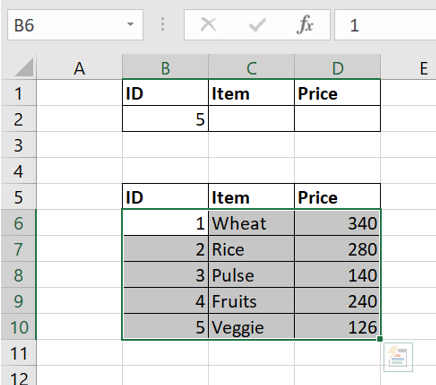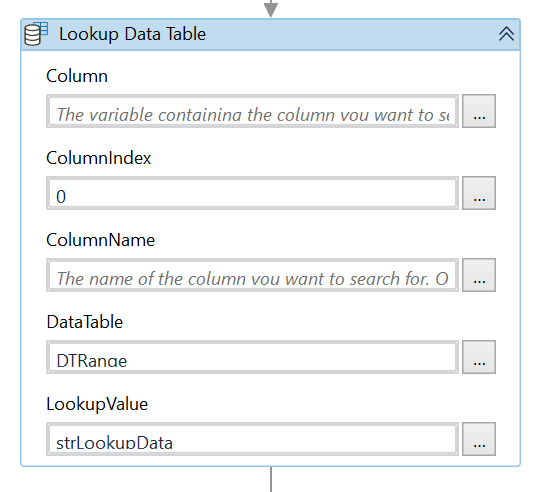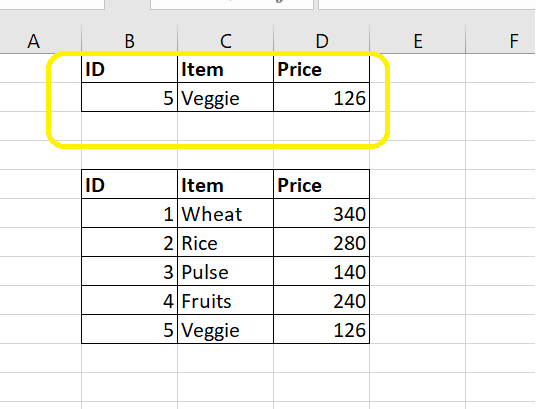# How to use Lookup DataTable in UiPath

In this tutorial we will learn about the vlookup function & its implementation in UiPath.

*Let’s refer the below excel data to lookup the Item & Price value.

We all aware about how to use the Vloookup function in Excel.

= vlookup (lookup value, table range, column index)

Now, we will use the same formula in UiPath to lookup the values.

Implementation in UiPath**

1. Open the Excel file in UiPath using “Excel Application Scope Activity”, store the excel location in the variable . Lets say strExcelLocation.
2. Read the range from the excel file using Read Range Activity from B6 to D10 & store it in a variable DTRange.3. Read the cell B2 from the file i.e lookup value & store it in a variable strLookupData.

4. Drag & drop Lookup Data Table activity & pass the values.

This lookup data table search the value of price in the table.Now, we will use another lookup data table to get the value for Item. The Only change would be in the Target Column.

5. Finally we will use write cell activity to write the data back to excel file at their specific location i.e C2 & D2Above are the variables & their types used in the process.

Let’s run the process now & check the result.I hope you enjoyed the article!
Happy Automation!!

Hello,

FYI - UiPath actually has a VLookup activity https://docs.uipath.com/activities/docs/v-lookup-x

Best regards,
Marius

1 Like